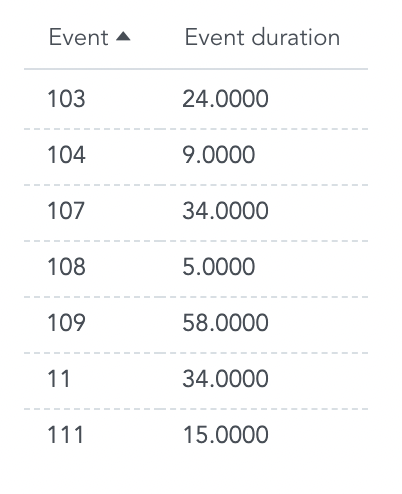# Finding Min and Max Dates Using Date Arithmetic

Sometimes you want to pull data based on the first or last dates when specific events occurred. For example, support agents want to uncover the oldest unsolved tickets in their help desk queue.

In GoodData, you can use date arithmetic with date attributes, the same logic can be applied in metrics, using less complex metrics and a simpler logical data model (LDM).

## Example LDM

To find out which type of event occurred first in each town, use date arithmetics to find the first, or MIN, Date (Start Date) that occur in each town.### Connecting Dates to Events

In the LDM diagram, facts and attributes can only be sliced and diced by objects directly connected to the left of them.

In this example, Date (Start Date) has no objects connected to its left.

So firstly, rejoin it to its connection point, Event, with MAQL:

``````SELECT Date (Start Date) BY Event
``````

The BY clause allows the metric to interact with the rest of the Event and Town datasets.

### Identifying the First (earliest) Date

Next, create a metric “First Start Date Per Town”. This finds the first Start Date that occurred in each Town:

``````SELECT MIN ((SELECT Date (Start Date) BY Event)) BY Town, ALL OTHER
``````

The BY Town and ALL OTHER causes that each town returns one date of the first event that occurred there, even if multiple events have occurred in each Town.

This date is represented by a numeric value and not an actual date:Tip: Use the following example to metric and custom number format to display date format as yyyy-mm-dd:

``````SELECT MAX ((SELECT (10000 * MAX(Year (Date))) + (100 * MAX(Month (Date))) + (MAX(Day of Month (Date))) BY <connection point>))
WHERE (SELECT COUNT(Date (Date), <connection point>) BY Year (Date), ALL OTHER) > 0
``````

Custom number format (see Formatting Numbers in Insights and Reports):

`````` [=null]N/A; ########
``````

### Inserting the Metric into Your Report

Lastly, add the previous metric into the final metric that finds the number of events that occurred on that specific date:

``````SELECT COUNT (Event) WHERE Date (Start Date) = First Start Date Per Town
``````

Use the “ First Start Date Per Town” metric to count only the first event that occurred in each town.

The final metric shows which Event and Type occurred first in each town. All subsequent Events have been filtered from the report.